# Lower Columbia River Fish Population Indexing Analysis 2017

The suggested citation for this analytic report is:

Thorley, J.L. (2018) Lower Columbia River Fish Population Indexing Analysis 2017. A Poisson Consulting Analysis Report. URL: https://www.poissonconsulting.ca/f/375247290.

## Background

In the mid 1990s BC Hydro began operating Hugh L. Keenleyside (HLK) Dam to reduce dewatering of Mountain Whitefish and Rainbow Trout eggs.

The primary goal of the Lower Columbia River Fish Population Indexing program is to answer two key management questions:

What are the abundance, growth rate, survival rate, body condition, age distribution, and spatial distribution of subadult and adult Whitefish, Rainbow Trout, and Walleye in the Lower Columbia River?

What is the effect of inter-annual variability in the Whitefish and Rainbow Trout flow regimes on the abundance, growth rate, survival rate, body condition, and spatial distribution of subadult and adult Whitefish, Rainbow Trout, and Walleye in the Lower Columbia River?

The inter-annual variability in the Whitefish and Rainbow Trout flow regimes was quantified in terms of the percent egg dewatering as greater flow variability is associated with more egg stranding.

## Methods

### Data Preparation

The fish indexing data were provided by Okanagan Nation Alliance and Golder Associates in the form of an Access database. The discharge and temperature data were obtained from the Columbia Basin Hydrological Database maintained by Poisson Consulting. The Rainbow Trout egg dewatering estimates were provided by CLBMON-46 (Irvine, Baxter, and Thorley 2015) and the Mountain Whitefish egg stranding estimates by Golder Associates (2013).

#### Discharge

Missing hourly discharge values for Hugh-Keenleyside Dam (HLK), Brilliant Dam (BRD) and Birchbank (BIR) were estimated by first leading the BIR values by 2 hours to account for the lag. Values missing at just one of the dams were then estimated assuming $$HLK + BRD = BIR$$. Negative values were set to be zero. Next, missing values spanning $$\leq$$ 28 days were estimated at HLK and BRD based on linear interpolation. Finally any remaining missing values at BIR were set to be $$HLK + BRD$$.

The data were prepared for analysis using R version 3.5.0 (R Core Team 2018).

### Data Analysis

Model parameters were estimated using hierarchical Bayesian methods. The parameters were produced using JAGS (Plummer 2015) and STAN (Carpenter et al. 2017). For additional information on Bayesian estimation the reader is referred to McElreath (2016).

The one exception is the length-at-age estimates which were produced using the mixdist R package (Macdonald 2012) which implements Maximum Likelihood with Expectation Maximization.

Unless indicated otherwise, the Bayesian analyses used normal and uniform prior distributions that were vague in the sense that they did not constrain the posteriors (Kery and Schaub 2011, 36). The posterior distributions were estimated from 1500 Markov Chain Monte Carlo (MCMC) samples thinned from the second halves of 3 chains (Kery and Schaub 2011, 38–40). Model convergence was confirmed by ensuring that $$\hat{R} \leq 1.05$$ (Kery and Schaub 2011, 40) and $$\textrm{ESS} \geq 150$$ for each of the monitored parameters (Kery and Schaub 2011, 61). Where $$\hat{R}$$ is the potential scale reduction factor and $$\textrm{ESS}$$ is the effective sample size (Brooks et al. 2011).

The parameters are summarised in terms of the point estimate, standard deviation (sd), the z-score, lower and upper 95% confidence/credible limits (CLs) and the p-value (Kery and Schaub 2011, 37, 42). For ML models, the point estimate is the MLE, the standard deviation is the standard error, the z-score is $$\mathrm{MLE}/\mathrm{sd}$$ and the 95% CLs are the $$\mathrm{MLE} \pm 1.96 \cdot \mathrm{sd}$$. For Bayesian models, the estimate is the median (50th percentile) of the MCMC samples, the z-score is $$\mathrm{mean}/\mathrm{sd}$$ and the 95% CLs are the 2.5th and 97.5th percentiles. A p-value of 0.05 indicates that the lower or upper 95% CL is 0.

Where relevant, model adequacy was confirmed by examination of residual plots for the full model(s).

The results are displayed graphically by plotting the modeled relationships between particular variables and the response(s) with the remaining variables held constant. In general, continuous and discrete fixed variables are held constant at their mean and first level values, respectively, while random variables are held constant at their typical values (expected values of the underlying hyperdistributions) (Kery and Schaub 2011, 77–82). When informative the influence of particular variables is expressed in terms of the effect size (i.e., percent change in the response variable) with 95% confidence/credible intervals (CIs, Bradford, Korman, and Higgins 2005).

The analyses were implemented using R version 3.5.0 (R Core Team 2018) and the mbr family of packages.

### Model Descriptions

#### Condition

The expected weight of fish of a given length were estimated from the data using a mass-length model (He et al. 2008). Key assumptions of the condition model include:

• The expected weight is allowed to vary with length and date.
• The expected weight is allowed to vary randomly with year.
• The relationship between weight and length is allowed to vary with date.
• The relationship between weight and length is allowed to vary randomly with year.
• The residual variation in weight is log-normally distributed.

Only previously untagged fish were included in models to avoid potential effects of tagging on body condition. Preliminary analyses indicated that the annual variation in weight was not correlated with the annual variation in the relationship between weight and length.

#### Growth

Annual growth of fish were estimated from the inter-annual recaptures using the Fabens method (Fabens 1965) for estimating the von Bertalanffy growth curve (von Bertalanffy 1938). This curve is based on the premise that:

$\frac{\text{d}L}{\text{d}t} = k (L_{\infty} - L)$

where $$L$$ is the length of the individual, $$k$$ is the growth coefficient and $$L_{\infty}$$ is the maximum length.

Integrating the above equation gives:

$L_t = L_{\infty} (1 - e^{-k(t - t_0)})$

where $$L_t$$ is the length at time $$t$$ and $$t_0$$ is the time at which the individual would have had zero length.

The Fabens form allows

$L_r = L_c + (L_{\infty} - L_c) (1 - e^{-kT})$

where $$L_r$$ is the length at recapture, $$L_c$$ is the length at capture and $$T$$ is the time between capture and recapture.

Key assumptions of the growth model include:

• The mean maximum length $$L_{\infty}$$ is constant.
• The growth coefficient $$k$$ is allowed to vary randomly with year.
• The residual variation in growth is normally distributed.

#### Movement

The extent to which sites are closed, i.e., fish remain at the same site between sessions, was evaluated with a logistic ANCOVA (Kery 2010). The model estimates the probability that intra-annual recaptures were caught at the same site versus a different one. Key assumptions of the site fidelity model include:

• The expected site fidelity is allowed to vary with fish length.
• Observed site fidelity is Bernoulli distributed.

Length as a second-order polynomial was not found to be a significant predictor for site fidelity.

#### Length-At-Age

The expected length-at-age of Mountain Whitefish and Rainbow Trout were estimated from annual length-frequency distributions using a finite mixture distribution model (Macdonald and Pitcher 1979)

There were assumed to be three distinguishable normally-distributed age-classes for Mountain Whitefish (Age-0, Age-1, Age-2 and Age-3+) two for Rainbow Trout (Age-0, Age-1, Age-2+). Initially the model was fitted to the data from all years combined. The model was then fitted to the data for each year separately with the initial values set to be the estimates from the combined values. The only constraints were that the standard deviations of the MW age-classes were identical in the combined analysis and fixed at the initial values in the individual years.

Rainbow Trout and Mountain Whitefish were categorized as Fry (Age-0), Juvenile (Age-1) and Adult (Age-2+) based on their length-based ages. All Walleye were considered to be Adults.

#### Survival

The annual adult survival rate was estimated by fitting a Cormack-Jolly-Seber model (Kery and Schaub 2011, 220–31) to inter-annual recaptures of adults.

Key assumptions of the survival model include:

• Survival varies randomly with year.
• The encounter probability for adults is allowed to vary with the total bank length sampled.

#### Observer Length Correction

The annual bias (inaccuracy) and error (imprecision) in observer’s fish length estimates were quantified from the divergence of the length distribution of their observed fish from the length distribution of the measured fish. More specifically, the percent length correction that minimised the Jensen-Shannon divergence (Lin 1991) between the two distributions provided a measure of the inaccuracy while the minimum divergence (the Jensen-Shannon divergence was calculated with log to base 2 which means it lies between 0 and 1) provided a measure of the imprecision.

#### Capture Efficiency

The probability of capture was estimated using a recapture-based binomial model (Kery and Schaub 2011, 134–36, 384–88).

Key assumptions of the capture efficiency model include:

• The capture probability varies randomly by session within year.
• The probability of a marked fish remaining at a site is the estimated site fidelity.
• The number of recaptures is described by a binomial distribution.

#### Abundance

The abundance was estimated from the catch and bias-corrected observer count data using an overdispersed Poisson model (Kery and Schaub 2011, 55–56).

Key assumptions of the abundance model include:

• The capture efficiency is the point estimate from the capture efficiency model.
• The efficiency varies by visit type (catch or count).
• The lineal fish density varies randomly with site, year and site within year.
• The overdispersion varies by visit type (count or catch).
• The catches and counts are described by a gamma-Poisson distribution.

#### Stock-Recruitment

The relationship between the adults and the resultant number of age-1 subadults was estimated using a Beverton-Holt stock-recruitment model (Walters and Martell 2004):

$R = \frac{\alpha \cdot S}{1 + \beta \cdot S} \quad,$

where $$S$$ is the adults (stock), $$R$$ is the subadults (recruits), $$\alpha$$ is the recruits per spawner at low density and $$\beta$$ determines the density-dependence.

Key assumptions of the stock-recruitment model include:

• The prior for $$\log(\alpha)$$ is a truncated normal distribution from $$\log(1)$$ to $$\log(5)$$.
• $$\beta$$ varies with the proportional egg loss.
• The residual variation in the number of recruits is log-normally distributed.

The carrying capacity $$K$$ is given by the relationship:

$K = \frac{\alpha}{\beta} \quad.$

#### Scale Age

The age of individual Mountain Whitefish was estimated from their circuli distances using a hand-coded algorithm. The algorithm involved a series of steps. First, the circuli distances were differenced to get the circuli increments. Next, a linear mixed model on the circuli number with the encounter as a random effect was fitted to the increments to account for differences in scale size and/or shape. A simple moving average (of window sma1) was then fitted to the residuals from the linear mixed model (circuli increment deviations) to remove noise. Small increment deviations, which are associated with annuli, were then identified by testing whether they are smaller than their neighbours based on a second simple moving average (of window sma2) and a multiplier. Finally blocks of small increment deviatons greater than a threshold size (block) were considered to be annuli. The initial circuli increment deviations below a second threshold were considered to have been laid down during the first year of life and were not included in the age (block count).

The algorithm was tuned by varying each of sma1, sma2, multiplier, block threshold and age-0 threshold within a narrow range of values and selecting the combination that minimized the age-averaged chi-squared statistic for encounters of known age. The age of an encounter was considered to be known if the length-at-age model was able to assign it to a single age-class with a probability $$\geq 0.98$$ or if the recaptures age was known at first capture.

#### Age-Ratios

The proportion of Age-1 Mountain Whitefish $$r^1_t$$ from a given spawn year $$t$$ is calculated from the relative abundance of Age-1 & Age-2 fish $$N^1_t$$ & $$N^2_t$$ respectively, which were lead or lagged so that all values were with respect to the spawn year:

$r^1_t = \frac{N^1_{t+2}}{N^1_{t+2} + N^2_{t+2}}$

The relative abundances of Age-1 and Age-2 fish were taken from the proportions of each age-class in the length-at-age analysis.

As the number of Age-2 fish might be expected to be influenced by the percentage egg loss $$Q_t$$ three years prior, the predictor variable $$\Pi_t$$ used is:

$\Pi_t = \textrm{log}(Q_t/Q_{t-1})$

The ratio was logged to ensure it was symmetrical about zero (Tornqvist, Vartia, and Vartia 1985).

The relationship between $$r^1_t$$ and $$\Pi_t$$ was estimated using a Bayesian regression (Kery 2010) loss model.

Key assumptions of the final model include:

• The log odds of the proportion of Age-1 fish varies with the log of the ratio of the percent egg losses.
• The residual variation is normally distributed.

The relationship between percent dewatering and subsequent recruitment is expected to depend on stock abundance (Subbey et al. 2014) which might be changing over the course of the study. Consequently, preliminary analyses allowed the slope of the regression line to change by year. However, year was not a significant predictor and was therefore removed from the final model. The effect of dewatering on Mountain Whitefish abundance was expressed in terms of the predicted percent change in Age-1 Mountain Whitefish abundance by egg loss in the spawn year relative to 10% egg loss in the spawn year. The egg loss in the previous year was fixed at 10%. The percent change could not be calculated relative to 0% in the spawn or previous year as $$\Pi_t$$ is undefined in either case.

### Condition

 data {
int nYear;
int nObs;

vector[nObs] Length;
vector[nObs] Weight;
vector[nObs] Dayte;
int Year[nObs];

parameters {
real bWeight;
real bWeightLength;
real bWeightDayte;
real bWeightLengthDayte;
real sWeightYear;
real sWeightLengthYear;

vector[nYear] bWeightYear;
vector[nYear] bWeightLengthYear;
real sWeight;

model {

vector[nObs] eWeight;

bWeight ~ normal(5, 5);
bWeightLength ~ normal(3, 2);

bWeightDayte ~ normal(0, 2);
bWeightLengthDayte ~ normal(0, 2);

sWeightYear ~ normal(0, 2);
sWeightLengthYear ~ normal(0, 2);

for (i in 1:nYear) {
bWeightYear[i] ~ normal(0, exp(sWeightYear));
bWeightLengthYear[i] ~ normal(0, exp(sWeightLengthYear));
}

sWeight ~ normal(0, 5);
for(i in 1:nObs) {
eWeight[i] = bWeight + bWeightDayte * Dayte[i] + bWeightYear[Year[i]] + (bWeightLength + bWeightLengthDayte * Dayte[i] + bWeightLengthYear[Year[i]]) * Length[i];
Weight[i] ~ lognormal(eWeight[i], exp(sWeight));
}
..

Template 1.

### Growth

model {
bK ~ dnorm (0, 5^-2)
sKYear ~ dnorm(0, 5^-2)

for (i in 1:nYear) {
bKYear[i] ~ dnorm(0, exp(sKYear)^-2)
log(eK[i]) <- bK + bKYear[i]
}

bLinf ~ dunif(100, 1000)
sGrowth ~ dnorm(0, 5^-2)
for (i in 1:length(Year)) {
eGrowth[i] <- (bLinf - LengthAtRelease[i]) * (1 - exp(-sum(eK[Year[i]:(Year[i] + dYears[i] - 1)])))
Growth[i] ~ dnorm(max(eGrowth[i], 0), exp(sGrowth)^-2)
}
..

Template 2.

### Movement

model {

bFidelity ~ dnorm(0, 2^-2)
bLength ~ dnorm(0, 2^-2)

for (i in 1:length(Fidelity)) {
logit(eFidelity[i]) <- bFidelity + bLength * Length[i]
Fidelity[i] ~ dbern(eFidelity[i])
}
..

Template 3.

### Survival

model{
bEfficiency ~ dnorm(0, 5^-2)
bEfficiencySampledLength ~ dnorm(0, 5^-2)

bSurvival ~ dnorm(0, 5^-2)

sSurvivalYear ~ dnorm(0, 5^-2)
for(i in 1:nYear) {
bSurvivalYear[i] ~ dnorm(0, exp(sSurvivalYear)^-2)
}

for(i in 1:(nYear-1)) {
logit(eEfficiency[i]) <- bEfficiency + bEfficiencySampledLength * SampledLength[i]
logit(eSurvival[i]) <- bSurvival + bSurvivalYear[i]

eProbability[i,i] <- eSurvival[i] * eEfficiency[i]
for(j in (i+1):(nYear-1)) {
eProbability[i,j] <- prod(eSurvival[i:j]) * prod(1-eEfficiency[i:(j-1)]) * eEfficiency[j]
}
for(j in 1:(i-1)) {
eProbability[i,j] <- 0
}
}
for(i in 1:(nYear-1)) {
eProbability[i,nYear] <- 1 - sum(eProbability[i,1:(nYear-1)])
}

for(i in 1:(nYear - 1)) {
Marray[i, 1:nYear] ~ dmulti(eProbability[i,], Released[i])
}
..

Template 4.

### Capture Efficiency

model {

bEfficiency ~ dnorm(0, 5^-2)

sEfficiencySessionAnnual ~ dnorm(0, 2^-2)
for (i in 1:nSession) {
for (j in 1:nAnnual) {
bEfficiencySessionAnnual[i, j] ~ dnorm(0, exp(sEfficiencySessionAnnual)^-2)
}
}

for (i in 1:length(Recaptures)) {

logit(eEfficiency[i]) <- bEfficiency + bEfficiencySessionAnnual[Session[i], Annual[i]]

eFidelity[i] ~ dnorm(Fidelity[i], FidelitySD[i]^-2) T(FidelityLower[i], FidelityUpper[i])
Recaptures[i] ~ dbin(eEfficiency[i] * eFidelity[i], Tagged[i])
}
..

Template 5.

### Abundance

model {
bDensity ~ dnorm(5, 5^-2)

sDensityAnnual ~ dnorm(0, 2^-2)
for (i in 1:nAnnual) {
bDensityAnnual[i] ~ dnorm(0, exp(sDensityAnnual)^-2)
}

sDensitySite ~ dnorm(0, 2^-2)
sDensitySiteAnnual ~ dnorm(0, 2^-2)
for (i in 1:nSite) {
bDensitySite[i] ~ dnorm(0, exp(sDensitySite)^-2)
for (j in 1:nAnnual) {
bDensitySiteAnnual[i, j] ~ dnorm(0, exp(sDensitySiteAnnual)^-2)
}
}

bEfficiencyVisitType <- 0
for (i in 2:nVisitType) {
bEfficiencyVisitType[i] ~ dnorm(0, 2^-2)
}

sDispersion ~ dnorm(0, 2^-2)
sDispersionVisitType <- 0
for(i in 2:nVisitType) {
sDispersionVisitType[i] ~ dnorm(0, 2^-2)
}

for (i in 1:length(Fish)) {
log(eDensity[i]) <- bDensity + bDensitySite[Site[i]] + bDensityAnnual[Annual[i]] + bDensitySiteAnnual[Site[i],Annual[i]]

eAbundance[i] <- eDensity[i] * SiteLength[i]

logit(eEfficiency[i]) <- logit(Efficiency[i]) + bEfficiencyVisitType[VisitType[i]]

log(esDispersion[i]) <- sDispersion + sDispersionVisitType[VisitType[i]]

eDispersion[i] ~ dgamma(esDispersion[i]^-2 + 0.1, esDispersion[i]^-2 + 0.1)
eFish[i] <- eAbundance[i] * ProportionSampled[i] * eEfficiency[i]
Fish[i] ~ dpois(eFish[i] * eDispersion[i])
}
..

Template 6.

### Stock-Recruitment

model {

bAlpha ~ dnorm(1, 2^-2) T(log(1), log(5))
bBeta ~ dnorm(-10, 5^-2)
bEggLoss ~ dnorm(0, 2^-2)

sRecruits ~ dnorm(0, 5^-2)
for(i in 1:length(Stock)){
log(eAlpha[i]) <- bAlpha
log(eBeta[i]) <- bBeta + bEggLoss * EggLoss[i]
eRecruits[i] <- (eAlpha[i] * Stock[i]) / (1 + eBeta[i] * Stock[i])
Recruits[i] ~ dlnorm(log(eRecruits[i]), exp(sRecruits)^-2)
}
..

Template 7.

### Age-Ratios

model{
bProbAge1 ~ dnorm(0, 2^-2)
bProbAge1Loss ~ dnorm(0, 2^-2)

sProbAge1 ~ dunif(0, 2)
for(i in 1:length(Age1Prop)){
eAge1Prop[i] <- bProbAge1 + bProbAge1Loss * LossLogRatio[i]
Age1Prop[i] ~ dnorm(eAge1Prop[i], sProbAge1^-2)
}
..

Template 8.

## Results

### Condition

Table 1. Parameter descriptions.

Parameter Description
bWeight Intercept of log(eWeight)
bWeightDayte Effect of Dayte on bWeight
bWeightLength Intercept of effect of Length on bWeight
bWeightLengthDayte Effect of Dayte on bWeightLength
bWeightLengthYear[i] Effect of ith Year on bWeightLength
bWeightYear[i] Effect of ith Year on bWeight
Dayte[i] Standardised day of year ith fish was captured
eWeight[i] Expected Weight of ith fish
Length[i] Log-transformed and centered fork length of ith fish
sWeight Log standard deviation of residual variation in log(Weight)
sWeightLengthYear Log standard deviation of bWeightLengthYear
sWeightYear Log standard deviation of bWeightYear
Weight[i] Recorded weight of ith fish
Year[i] Year ith fish was captured

#### Mountain Whitefish

Table 2. Model coefficients.

term estimate sd zscore lower upper pvalue
bWeight 5.4294212 0.0104229 520.916540 5.4097270 5.4492954 0.0007
bWeightDayte -0.0159965 0.0019319 -8.273691 -0.0197489 -0.0122547 0.0007
bWeightLength 3.1589240 0.0248477 127.123133 3.1085492 3.2068216 0.0007
bWeightLengthDayte -0.0078296 0.0052733 -1.450593 -0.0176503 0.0026358 0.1547
sWeight -1.9074108 0.0061874 -308.315251 -1.9192942 -1.8957215 0.0007
sWeightLengthYear -2.2877071 0.2067677 -11.032237 -2.6563198 -1.8565276 0.0007
sWeightYear -3.1270272 0.1756062 -17.766849 -3.4643990 -2.7675448 0.0007

Table 3. Model summary.

n K nchains niters nthin ess rhat converged
13422 7 3 500 2 358 1.006 TRUE

#### Rainbow Trout

Table 4. Model coefficients.

term estimate sd zscore lower upper pvalue
bWeight 5.9791123 0.0059613 1002.959006 5.9662992 5.9905083 0.0007
bWeightDayte -0.0037758 0.0013122 -2.902873 -0.0064961 -0.0013717 0.0013
bWeightLength 2.9255780 0.0118616 246.595286 2.9008210 2.9479510 0.0007
bWeightLengthDayte 0.0384326 0.0039495 9.755974 0.0308850 0.0463159 0.0007
sWeight -2.2538561 0.0062821 -358.769320 -2.2664328 -2.2413489 0.0007
sWeightLengthYear -3.0158107 0.1961825 -15.327172 -3.3700589 -2.5985948 0.0007
sWeightYear -3.6355060 0.1671642 -21.687819 -3.9169343 -3.2706035 0.0007

Table 5. Model summary.

n K nchains niters nthin ess rhat converged
13671 7 3 500 1 234 1.005 TRUE

#### Walleye

Table 6. Model coefficients.

term estimate sd zscore lower upper pvalue
bWeight 6.2898298 0.0077988 806.505412 6.2742873 6.3055566 0.0007
bWeightDayte 0.0163324 0.0014428 11.331798 0.0134977 0.0191421 0.0007
bWeightLength 3.2298520 0.0209100 154.475415 3.1867992 3.2727126 0.0007
bWeightLengthDayte -0.0132222 0.0086535 -1.541439 -0.0309711 0.0033237 0.1053
sWeight -2.3651037 0.0075800 -312.016650 -2.3795661 -2.3498578 0.0007
sWeightLengthYear -2.5023394 0.1986423 -12.570806 -2.8826675 -2.0771975 0.0007
sWeightYear -3.3033678 0.1720936 -19.149392 -3.5926969 -2.9368921 0.0007

Table 7. Model summary.

n K nchains niters nthin ess rhat converged
8876 7 3 500 1 238 1.011 TRUE

### Growth

Table 8. Parameter descriptions.

Parameter Description
bK Intercept of log(eK)
bKYear[i] Effect of ith Year on bK
bLinf Mean maximum length
dYears[i] Years between release and recapture of ith recapture
eGrowth Expected Growth between release and recapture
eK[i] Expected von Bertalanffy growth coefficient from i-1th to ith year
Growth[i] Observed growth between release and recapture of ith recapture
LengthAtRelease[i] Length at previous release of ith recapture
sGrowth Log standard deviation of residual variation in Growth
sKYear Log standard deviation of bKYear
Year[i] Release year of ith recapture

#### Mountain Whitefish

Table 9. Model coefficients.

term estimate sd zscore lower upper pvalue
bK -0.8886555 0.1124476 -7.929429 -1.124709 -0.6700452 0.0007
bLinf 392.0554701 3.3943312 115.560885 385.992525 399.4185467 0.0007
sGrowth 2.5056451 0.0473208 52.955425 2.416305 2.6015461 0.0007
sKYear -1.1074394 0.2848052 -3.885767 -1.671213 -0.5295446 0.0013

Table 10. Model summary.

n K nchains niters nthin ess rhat converged
234 4 3 500 20 627 1.004 TRUE

#### Rainbow Trout

Table 11. Model coefficients.

term estimate sd zscore lower upper pvalue
bK -0.1652507 0.0734300 -2.249802 -0.3133925 -0.0134686 0.0333
bLinf 490.0335024 2.9089229 168.464706 484.4790208 495.7201166 0.0007
sGrowth 3.3786477 0.0216482 156.096301 3.3375430 3.4225138 0.0007
sKYear -1.3629170 0.1930054 -7.025804 -1.7035313 -0.9577791 0.0007

Table 12. Model summary.

n K nchains niters nthin ess rhat converged
1061 4 3 500 20 477 1.004 TRUE

#### Walleye

Table 13. Model coefficients.

term estimate sd zscore lower upper pvalue
bK -2.549841 0.2642482 -9.675154 -3.044665 -2.058332 0.0007
bLinf 751.584259 88.5302441 8.648111 626.812966 960.982689 0.0007
sGrowth 2.879025 0.0468696 61.413981 2.789731 2.969387 0.0007
sKYear -1.105929 0.2687935 -4.093901 -1.608352 -0.569811 0.0013

Table 14. Model summary.

n K nchains niters nthin ess rhat converged
254 4 3 500 40 184 1.005 TRUE

### Movement

Table 15. Parameter descriptions.

Parameter Description
bFidelity Intercept of logit(eFidelity)
bLength Effect of length on logit(eFidelity)
eFidelity[i] Expected site fidelity of ith recapture
Fidelity[i] Whether the ith recapture was encountered at the same site as the previous encounter
Length[i] Length at previous encounter of ith recapture

#### Mountain Whitefish

Table 16. Model coefficients.

term estimate sd zscore lower upper pvalue
bFidelity -0.1681327 0.1917864 -0.8653585 -0.5569344 0.2141905 0.3947
bLength -0.0989941 0.1850241 -0.5236471 -0.4525534 0.2541279 0.6133

Table 17. Model summary.

n K nchains niters nthin ess rhat converged
115 2 3 500 1 963 1.004 TRUE

#### Rainbow Trout

Table 18. Model coefficients.

term estimate sd zscore lower upper pvalue
bFidelity 0.7971779 0.0787477 10.135053 0.6500068 0.9494468 7e-04
bLength -0.3188989 0.0790255 -4.093261 -0.4815628 -0.1772360 7e-04

Table 19. Model summary.

n K nchains niters nthin ess rhat converged
727 2 3 500 1 782 1.005 TRUE

#### Walleye

Table 20. Model coefficients.

term estimate sd zscore lower upper pvalue
bFidelity 0.688804 0.1433271 4.8394646 0.4161226 0.9739216 7e-04
bLength -0.035624 0.1451421 -0.2579797 -0.3346848 0.2363574 8e-01

Table 21. Model summary.

n K nchains niters nthin ess rhat converged
216 2 3 500 1 788 1 TRUE

### Length-At-Age

#### Mountain Whitefish

Table 22. The estimated upper length cutoffs (mm) by age and year.

Year Age0 Age1 Age2
1990 159 253 288
1991 144 226 297
2001 141 257 343
2002 163 260 343
2003 160 263 353
2004 158 249 342
2005 168 263 362
2006 175 284 356
2007 171 279 338
2008 170 248 341
2009 169 265 355
2010 177 272 353
2011 163 269 349
2012 162 268 347
2013 185 282 350
2014 178 283 362
2015 167 277 366
2016 165 282 352
2017 158 269 354

#### Rainbow Trout

Table 23. The estimated upper length cutoffs (mm) by age and year.

Year Age0 Age1
1990 155 358
1991 127 343
2001 133 324
2002 154 350
2003 161 343
2004 142 333
2005 164 347
2006 170 365
2007 166 375
2008 146 340
2009 147 339
2010 143 337
2011 156 344
2012 152 344
2013 169 355
2014 154 337
2015 167 334
2016 154 337
2017 133 317

### Survival

Table 24. Parameter descriptions.

Parameter Description
bEfficiency Intercept for logit(eEfficiency)
bEfficiencySampledLength Effect of SampledLength on bEfficiency
bSurvival Intercept for logit(eSurvival)
bSurvivalYear[i] Effect of Year on bSurvival
eEfficiency[i] Expected recapture probability in ith year
eSurvival[i] Expected survival probability from i-1th to ith year
SampledLength Total standardised length of river sampled
sSurvivalYear Log SD of bSurvivalYear

#### Mountain Whitefish

Table 25. Model coefficients.

term estimate sd zscore lower upper pvalue
bEfficiency -4.2446253 0.1159913 -36.624695 -4.4823947 -4.0346703 0.0007
bEfficiencySampledLength 0.4838203 0.1289041 3.762524 0.2397391 0.7548392 0.0007
bSurvival 0.9930812 0.4906920 2.111555 0.1403570 2.1629080 0.0240
sSurvivalYear 0.4267963 0.3395373 1.247323 -0.2306143 1.0868005 0.2067

Table 26. Model summary.

n K nchains niters nthin ess rhat converged
16 4 3 500 20 309 1.011 TRUE

#### Rainbow Trout

Table 27. Model coefficients.

term estimate sd zscore lower upper pvalue
bEfficiency -2.5152846 0.0950245 -26.4849508 -2.7066001 -2.3324168 0.0007
bEfficiencySampledLength 0.0095463 0.0781437 0.1393196 -0.1381551 0.1688784 0.8933
bSurvival -0.4648874 0.1244426 -3.7236758 -0.7057194 -0.2139384 0.0007
sSurvivalYear -1.1833806 0.7035404 -1.8780897 -3.3992595 -0.5055175 0.0007

Table 28. Model summary.

n K nchains niters nthin ess rhat converged
16 4 3 500 20 356 1.013 TRUE

#### Walleye

Table 29. Model coefficients.

term estimate sd zscore lower upper pvalue
bEfficiency -3.3746728 0.1066350 -31.6284314 -3.5822998 -3.1651352 0.0007
bEfficiencySampledLength 0.0722225 0.0908089 0.8042139 -0.0995937 0.2536344 0.4253
bSurvival 0.0752269 0.1561675 0.5612343 -0.1905603 0.4284746 0.5893
sSurvivalYear -0.9238995 1.0595874 -1.1130358 -4.5277896 -0.1806713 0.0173

Table 30. Model summary.

n K nchains niters nthin ess rhat converged
16 4 3 500 40 254 1.033 TRUE

### Capture Efficiency

Table 31. Parameter descriptions.

Parameter Description
Annual[i] Year of ith visit
bEfficiency Intercept for logit(eEfficiency)
bEfficiencySessionAnnual Effect of Session within Annual on logit(eEfficiency)
eEfficiency[i] Expected efficiency on ith visit
eFidelity[i] Expected site fidelity on ith visit
Fidelity[i] Mean site fidelity on ith visit
FidelitySD[i] SD of site fidelity on ith visit
Recaptures[i] Number of marked fish recaught during ith visit
sEfficiencySessionAnnual Log SD of effect of Session within Annual on logit(eEfficiency)
Session[i] Session of ith visit
Tagged[i] Number of marked fish tagged prior to ith visit

#### Mountain Whitefish

Table 32. Model coefficients.

term estimate sd zscore lower upper pvalue
bEfficiency -4.941610 0.2182581 -22.726014 -5.459583 -4.6046780 0.0007
sEfficiencySessionAnnual -1.023467 1.1297632 -1.162838 -4.219376 0.0912245 0.0907

Table 33. Model summary.

n K nchains niters nthin ess rhat converged
1299 2 3 500 100 217 1.017 TRUE

Table 34. Model coefficients.

term estimate sd zscore lower upper pvalue
bEfficiency -5.207899 0.1490366 -34.97314 -5.519861 -4.9309168 7e-04
sEfficiencySessionAnnual -1.992512 1.1615237 -1.91703 -5.088239 -0.6557197 7e-04

Table 35. Model summary.

n K nchains niters nthin ess rhat converged
1468 2 3 500 100 237 1.014 TRUE

#### Rainbow Trout

Table 36. Model coefficients.

term estimate sd zscore lower upper pvalue
bEfficiency -3.3845947 0.0663431 -51.009127 -3.511163 -3.2539976 7e-04
sEfficiencySessionAnnual -0.9316827 0.1633991 -5.746617 -1.283852 -0.6348329 7e-04

Table 37. Model summary.

n K nchains niters nthin ess rhat converged
1487 2 3 500 50 1278 1.004 TRUE

Table 38. Model coefficients.

term estimate sd zscore lower upper pvalue
bEfficiency -4.031955 0.0686749 -58.707182 -4.169615 -3.905716 7e-04
sEfficiencySessionAnnual -2.003418 0.8916414 -2.486169 -4.397031 -1.049144 7e-04

Table 39. Model summary.

n K nchains niters nthin ess rhat converged
1545 2 3 500 100 252 1.015 TRUE

#### Walleye

Table 40. Model coefficients.

term estimate sd zscore lower upper pvalue
bEfficiency -4.5514548 0.1248230 -36.509723 -4.835597 -4.3221467 7e-04
sEfficiencySessionAnnual -0.5398251 0.2158204 -2.548474 -1.003360 -0.1761849 4e-03

Table 41. Model summary.

n K nchains niters nthin ess rhat converged
1601 2 3 500 50 1224 1.005 TRUE

### Abundance

Table 42. Parameter descriptions.

Parameter Description
Annual Year
bDensity Intercept for log(eDensity)
bDensityAnnual Effect of Annual on bDensity
bDensitySite Effect of Site on bDensity
bDensitySiteAnnual Effect of Site within Annual on bDensity
bEfficiencyVisitType Effect of VisitType on Efficiency
eDensity Expected density
Efficiency Capture efficiency
esDispersion Overdispersion of Fish
Fish Number of fish captured or counted
ProportionSampled Proportion of site surveyed
sDensityAnnual Log SD of effect of Annual on bDensity
sDensitySite Log SD of effect of Site on bDensity
sDensitySiteAnnual Log SD of effect of Site within Annual on bDensity
sDispersion Intercept for log(esDispersion)
sDispersionVisitType Effect of VisitType on sDispersion
Site Site
SiteLength Length of site
VisitType Survey type (catch versus count)

#### Mountain Whitefish

Table 43. Model coefficients.

term estimate sd zscore lower upper pvalue
bDensity 5.4765903 0.1861413 29.407587 5.1026572 5.8323686 0.0007
bEfficiencyVisitType 1.4860960 0.0801141 18.548050 1.3344746 1.6503053 0.0007
sDensityAnnual -0.3789589 0.1843361 -2.004608 -0.7057124 0.0122872 0.0587
sDensitySite -0.3014650 0.1053811 -2.867956 -0.5094493 -0.0862499 0.0013
sDensitySiteAnnual -0.8258834 0.0629434 -13.143671 -0.9504982 -0.7041190 0.0007
sDispersion -0.7542474 0.0458290 -16.472246 -0.8469796 -0.6673892 0.0007
sDispersionVisitType 0.5072713 0.0949927 5.354164 0.3241520 0.6973576 0.0007

Table 44. Model summary.

n K nchains niters nthin ess rhat converged
2391 7 3 500 200 219 1.008 TRUE

Table 45. Model coefficients.

term estimate sd zscore lower upper pvalue
bDensity 6.2920251 0.1661460 37.906648 5.9587938 6.6104837 0.0007
bEfficiencyVisitType 1.8182092 0.0898705 20.266968 1.6446940 1.9949233 0.0007
sDensityAnnual -1.0424453 0.2058076 -5.020928 -1.4065557 -0.6015453 0.0007
sDensitySite 0.1118483 0.0975265 1.188015 -0.0766389 0.3110734 0.2227
sDensitySiteAnnual -0.9021927 0.0648302 -13.923568 -1.0276214 -0.7742620 0.0007
sDispersion -0.6333482 0.0345890 -18.301069 -0.7020864 -0.5656565 0.0007
sDispersionVisitType 0.5364494 0.0836070 6.381720 0.3701216 0.6951912 0.0007

Table 46. Model summary.

n K nchains niters nthin ess rhat converged
2391 7 3 500 100 156 1.019 TRUE

#### Rainbow Trout

Table 47. Model coefficients.

term estimate sd zscore lower upper pvalue
bDensity 4.8482784 0.1101237 44.002798 4.6164097 5.0487444 7e-04
bEfficiencyVisitType 1.5145148 0.0794431 19.108376 1.3721645 1.6754129 7e-04
sDensityAnnual -1.2885872 0.2095606 -6.138977 -1.6901852 -0.8703518 7e-04
sDensitySite -0.3538945 0.0995714 -3.547526 -0.5445650 -0.1626558 7e-04
sDensitySiteAnnual -0.8838403 0.0560701 -15.788300 -0.9980944 -0.7810704 7e-04
sDispersion -0.9522129 0.0415707 -22.955099 -1.0363156 -0.8755609 7e-04
sDispersionVisitType 0.5865835 0.0946903 6.176692 0.3967560 0.7602344 7e-04

Table 48. Model summary.

n K nchains niters nthin ess rhat converged
2391 7 3 500 100 260 1.007 TRUE

Table 49. Model coefficients.

term estimate sd zscore lower upper pvalue
bDensity 5.4427109 0.1195159 45.505604 5.1951437 5.6676320 7e-04
bEfficiencyVisitType 1.3055565 0.0657781 19.877116 1.1741961 1.4379650 7e-04
sDensityAnnual -1.1997397 0.1936541 -6.152570 -1.5440803 -0.7983087 7e-04
sDensitySite -0.4379821 0.0975582 -4.437252 -0.6142232 -0.2303591 7e-04
sDensitySiteAnnual -1.2203696 0.0729850 -16.774980 -1.3683662 -1.0880160 7e-04
sDispersion -1.0144820 0.0475458 -21.354124 -1.1164392 -0.9266665 7e-04
sDispersionVisitType 0.5819554 0.0962208 6.050765 0.3978520 0.7699114 7e-04

Table 50. Model summary.

n K nchains niters nthin ess rhat converged
2391 7 3 500 100 251 1.012 TRUE

#### Walleye

Table 51. Model coefficients.

term estimate sd zscore lower upper pvalue
bDensity 5.4307042 0.1258833 43.106649 5.1724770 5.6656843 7e-04
bEfficiencyVisitType 1.2318520 0.0802977 15.329270 1.0713218 1.3844783 7e-04
sDensityAnnual -0.8157032 0.1843167 -4.421948 -1.1569998 -0.4333404 7e-04
sDensitySite -1.0547288 0.1435021 -7.377644 -1.3425560 -0.7928187 7e-04
sDensitySiteAnnual -1.3424684 0.0924602 -14.518714 -1.5360284 -1.1713057 7e-04
sDispersion -0.8126212 0.0400443 -20.305996 -0.8936627 -0.7371643 7e-04
sDispersionVisitType 0.5085584 0.1009985 5.039459 0.3083867 0.6986985 7e-04

Table 52. Model summary.

n K nchains niters nthin ess rhat converged
2391 7 3 500 100 249 1.015 TRUE

### Stock-Recruitment

Table 53. Parameter descriptions.

Parameter Description
bAlpha Intercept for log(eAlpha)
bBeta Intercept for log(eBeta)
bEggLoss Effect of EggLoss on bBeta
eAlpha eRecruits per Stock at low Stock density
eBeta Expected density-dependence
EggLoss Calculated proportional egg loss
eRecruits Expected Recruits
Recruits Number of Age-1 recruits
sRecruits Log SD of residual variation in Recruits
Stock Number of Age-2+ spawners

#### Mountain Whitefish

Table 54. Model coefficients.

term estimate sd zscore lower upper pvalue
bAlpha 0.8139611 0.4446271 1.8384688 0.0695496 1.5512923 0.0007
bBeta -9.7597367 0.5537169 -17.6694263 -10.8393829 -8.8183202 0.0007
bEggLoss 0.0860254 0.2179828 0.3908444 -0.3726391 0.5233172 0.6787
sRecruits -0.4619553 0.2177265 -2.0973882 -0.8410797 0.0023342 0.0533

Table 55. Model summary.

n K nchains niters nthin ess rhat converged
15 4 3 500 50 1252 1.001 TRUE

#### Rainbow Trout

Table 56. Model coefficients.

term estimate sd zscore lower upper pvalue
bAlpha 0.9040279 0.4368077 2.011898 0.0715993 1.5797639 0.0007
bBeta -9.3898676 0.6571494 -14.431341 -10.8339241 -8.5409575 0.0007
bEggLoss -0.2074621 0.1598935 -1.485111 -0.6006027 -0.0320101 0.0240
sRecruits -1.4767867 0.2035237 -7.211967 -1.8300063 -1.0273612 0.0007

Table 57. Model summary.

n K nchains niters nthin ess rhat converged
16 4 3 500 50 310 1.004 TRUE

### Scale Age

Table 58. The optimal parameter values for aging fish from their circuli distances.

sma1 sma2 multiplier block age0
3 12 0.2 4 40

### Age-Ratios

Table 59. Parameter descriptions.

Parameter Description
Age1[i] The number of Age-1 fish in the ith year
Age1and2[i] The number of Age-1 and Age-2 fish in the ith year
bProbAge1 Intercept for logit(eProbAge1)
bProbAge1Loss Effect of LossLogRatio on bProbAge1
eProbAge1[i] The expected proportion of Age-1 fish in the ith year
LossLogRatio[i] The log of the ratio of the percent egg losses
sDispersion SD of extra-binomial variation

Table 60. Model coefficients.

term estimate sd zscore lower upper pvalue
bProbAge1 0.2764236 0.2186040 1.2242454 -0.1768765 0.6933735 0.1960
bProbAge1Loss -0.2193412 0.3303882 -0.6362767 -0.8510240 0.4972314 0.4773
sProbAge1 0.8401563 0.1967772 4.4300797 0.5952813 1.3401481 0.0007

Table 61. Model summary.

n K nchains niters nthin ess rhat converged
17 3 3 500 1 471 1.003 TRUE

### Condition

##### Mountain Whitefish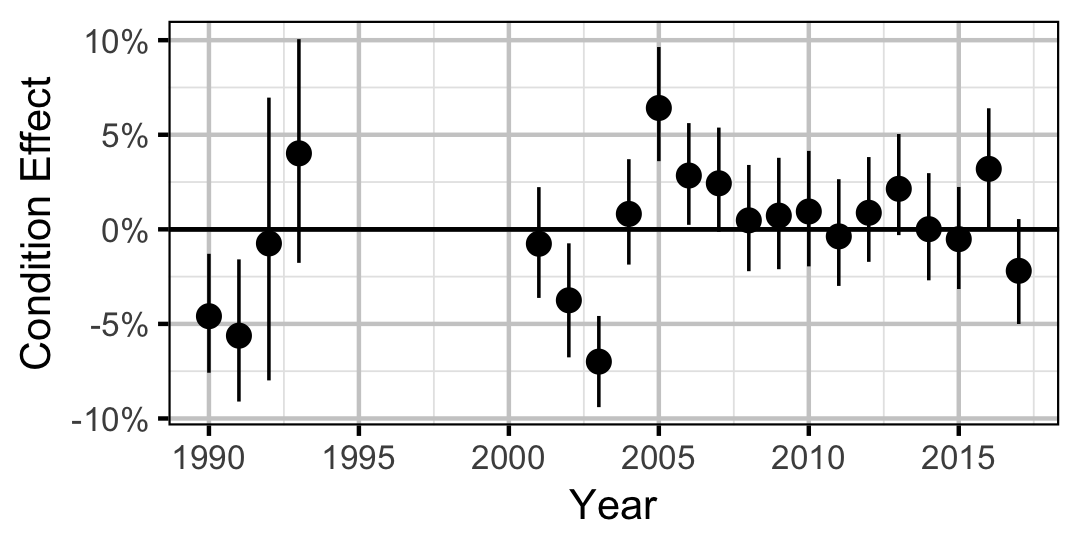Figure 2. Estimated change in condition relative to a typical year for a 200 mm Mountain Whitefish by year (with 95% CRIs).
##### Rainbow Trout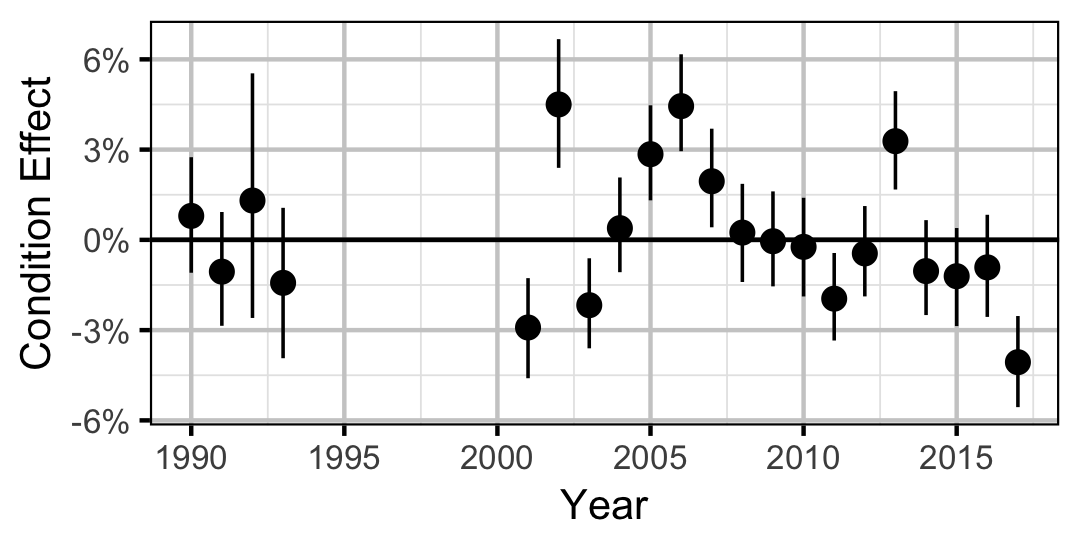Figure 3. Estimated change in condition relative to a typical year for a 250 mm Rainbow Trout by year (with 95% CRIs).

##### Mountain Whitefish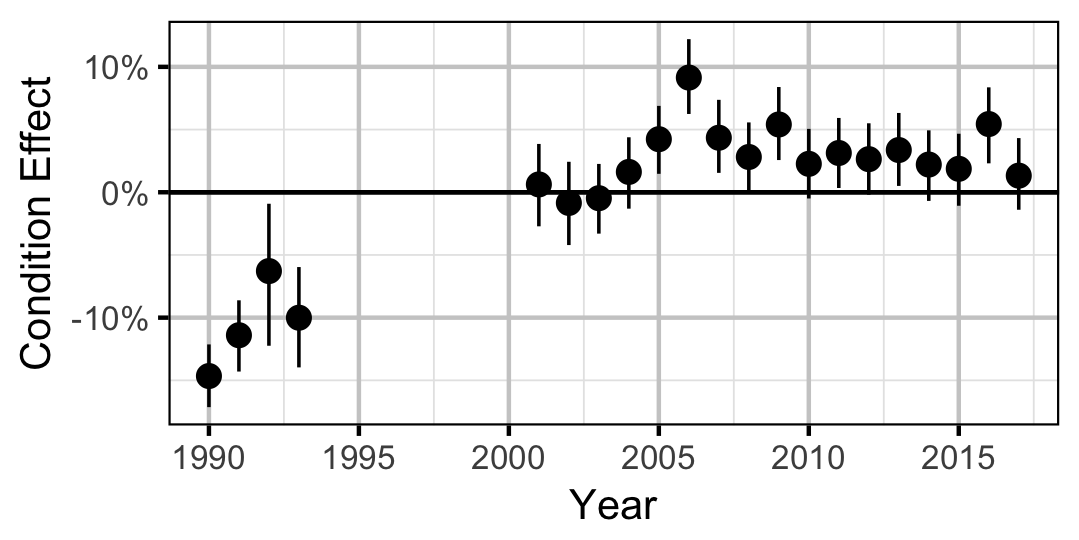Figure 4. Estimated change in condition relative to a typical year for a 350 mm Mountain Whitefish by year (with 95% CRIs).
##### Rainbow Trout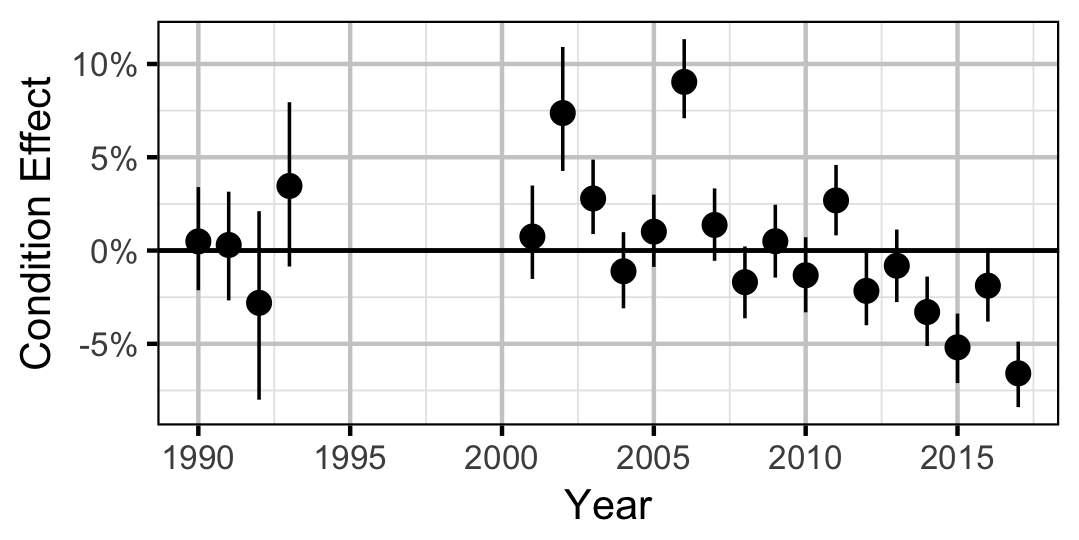Figure 5. Estimated change in condition relative to a typical year for a 500 mm Rainbow Trout by year (with 95% CRIs).
##### Walleye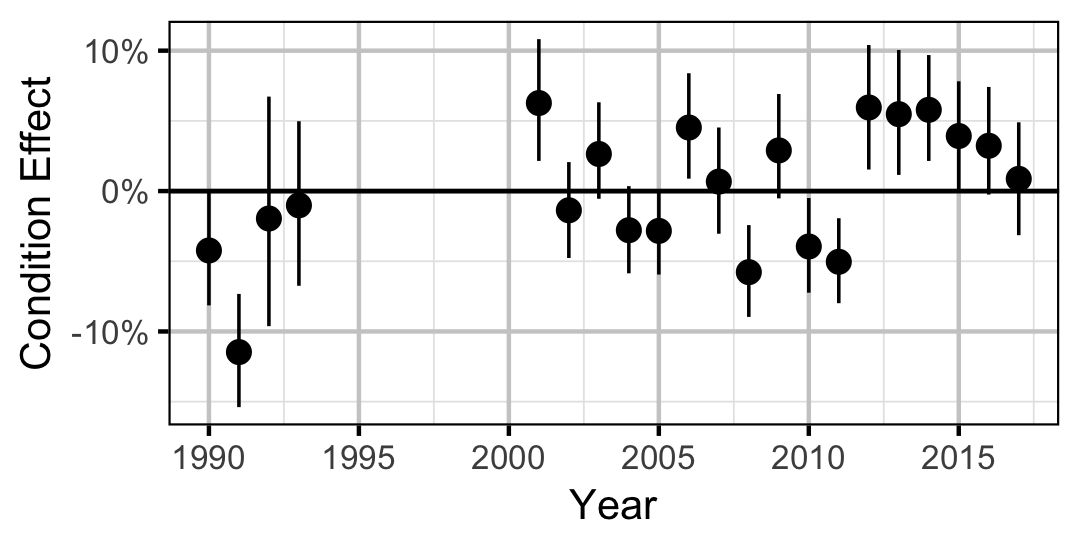Figure 6. Estimated change in condition relative to a typical year for a 600 mm Walleye by year (with 95% CRIs).

### Growth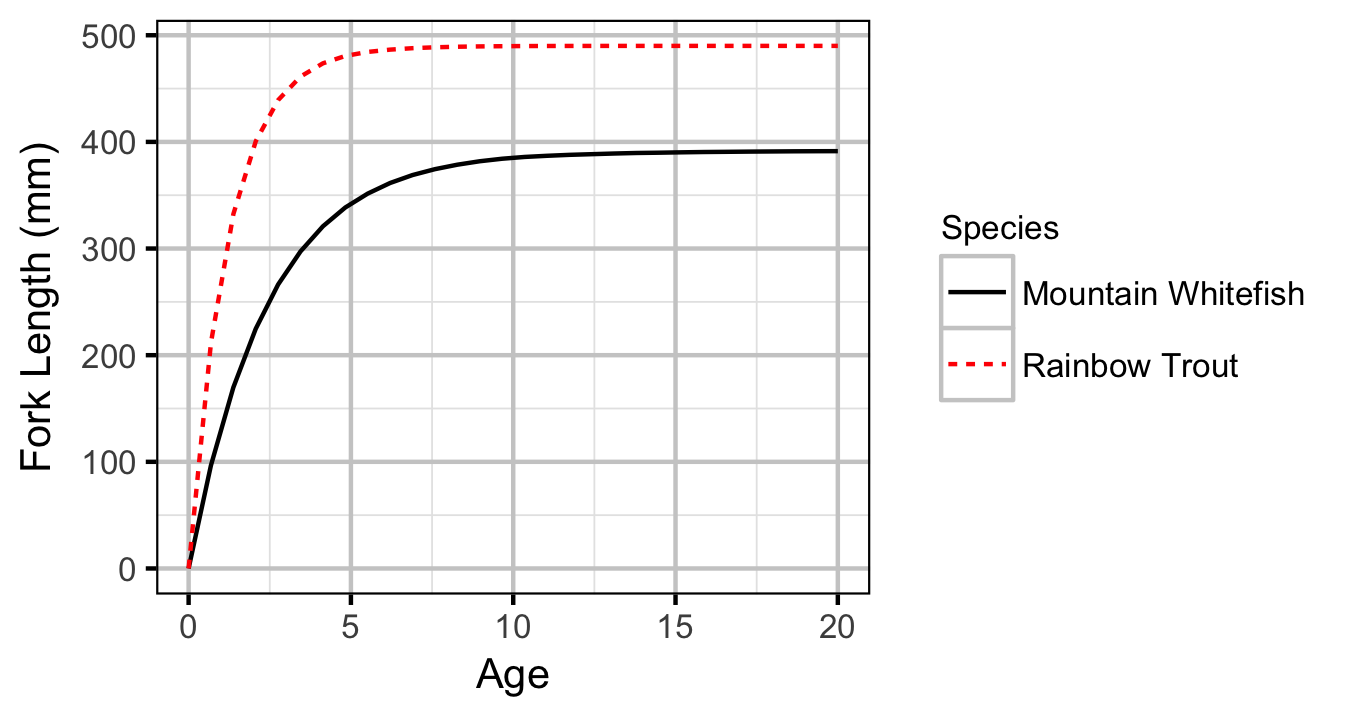Figure 7. Estimated von Bertalanffy growth curve by species. The growth curve for Walleye is not shown because the growth parameters were not representative for the younger age-classes.

#### Mountain Whitefish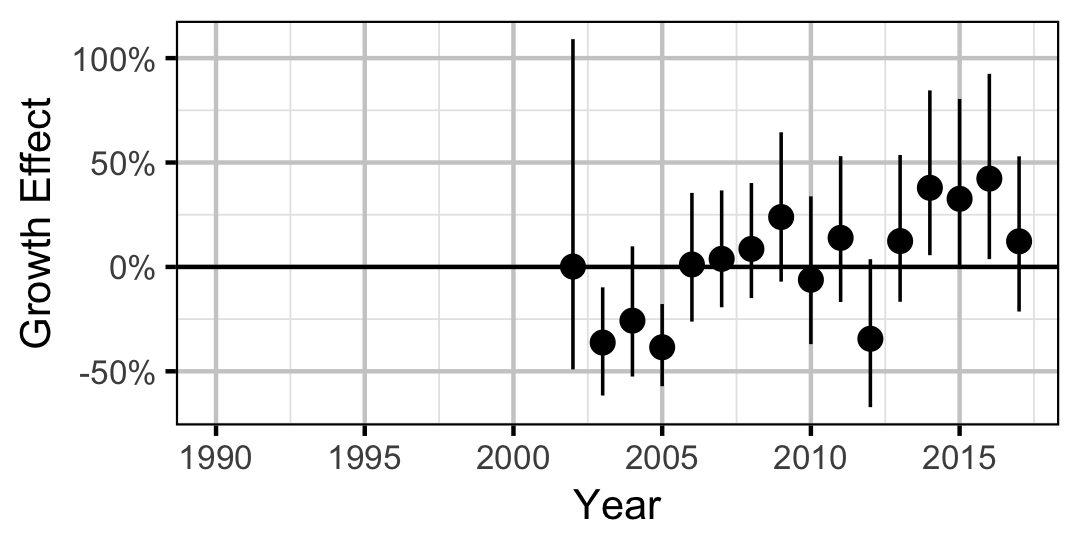Figure 8. Estimated change in von Bertalanffy growth coefficient (k) relative to a typical year by year (with 95% CIs).

#### Rainbow Trout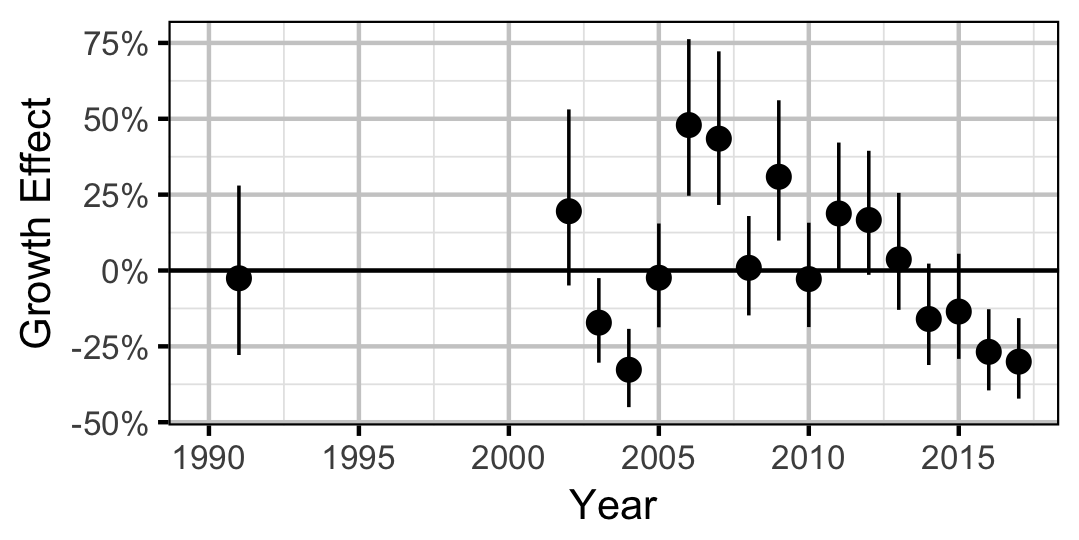Figure 9. Estimated change in von Bertalanffy growth coefficient (k) relative to a typical year by year (with 95% CIs).

#### Walleye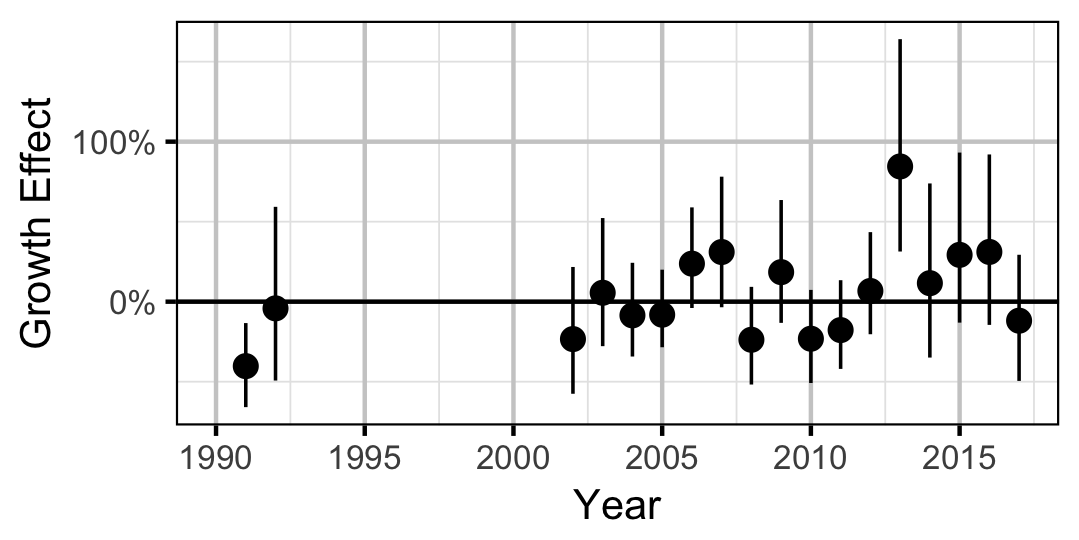Figure 10. Estimated change in von Bertalanffy growth coefficient (k) relative to a typical year by year (with 95% CIs).

### Movement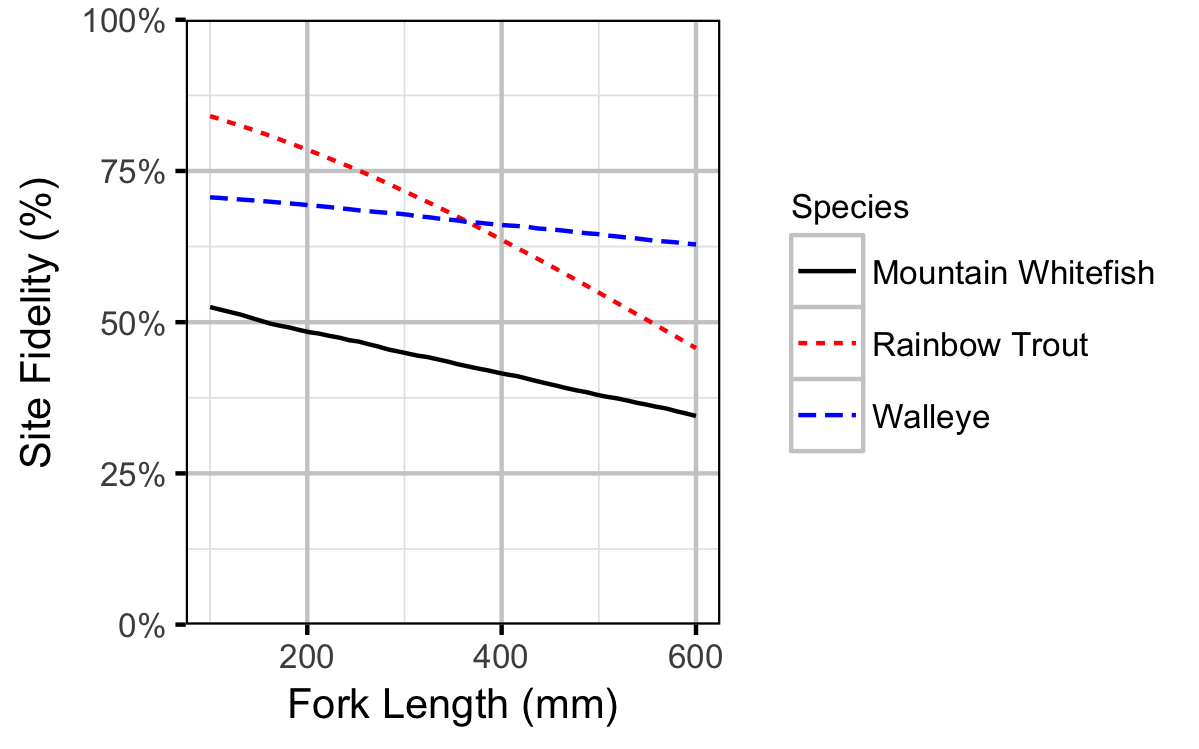Figure 11. Probability of recapture at the same site versus a different site by fish length (with 95% CRIs).

### Observer Length Correction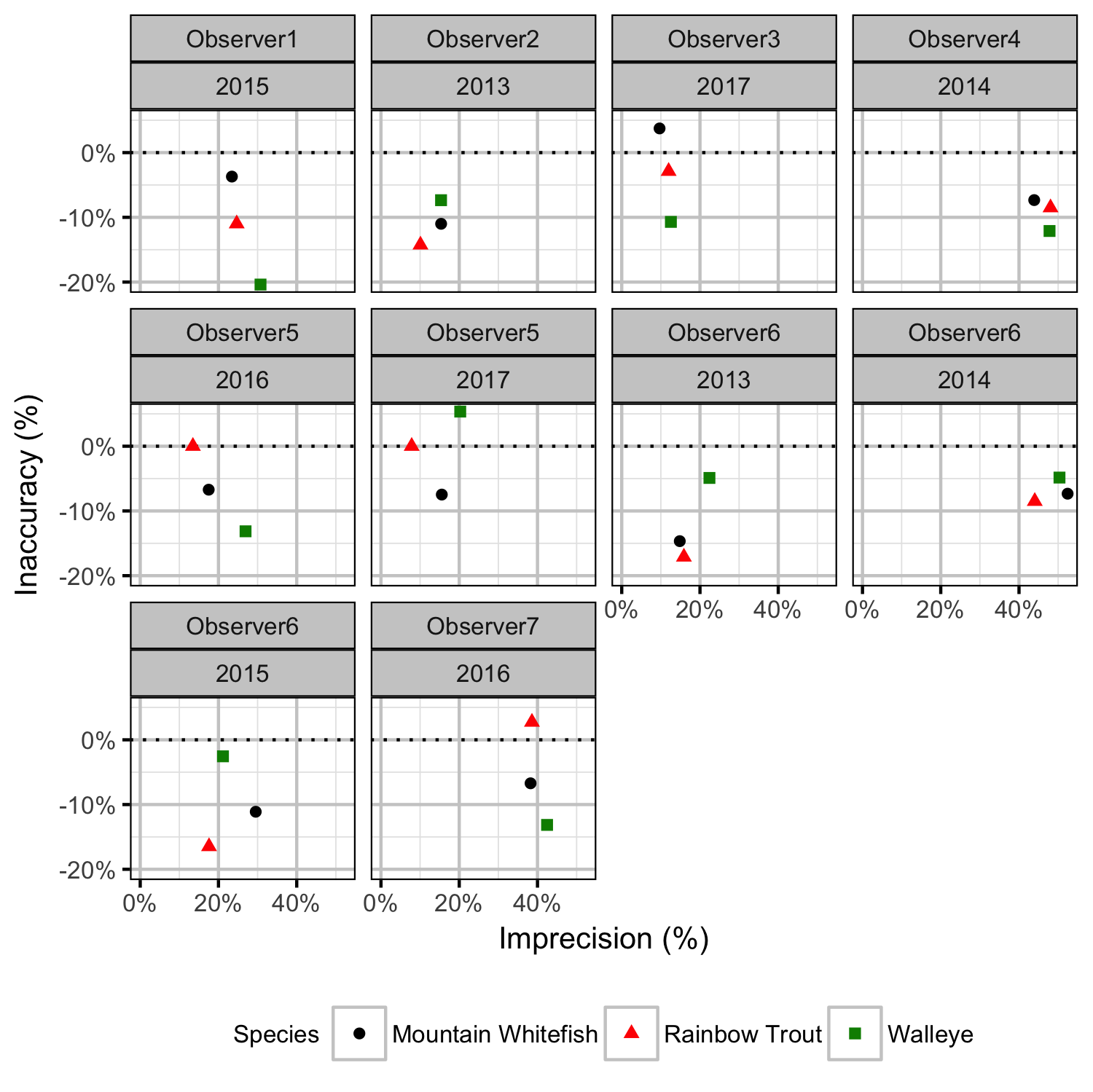Figure 22. Length inaccuracy and imprecision by observer, year and species.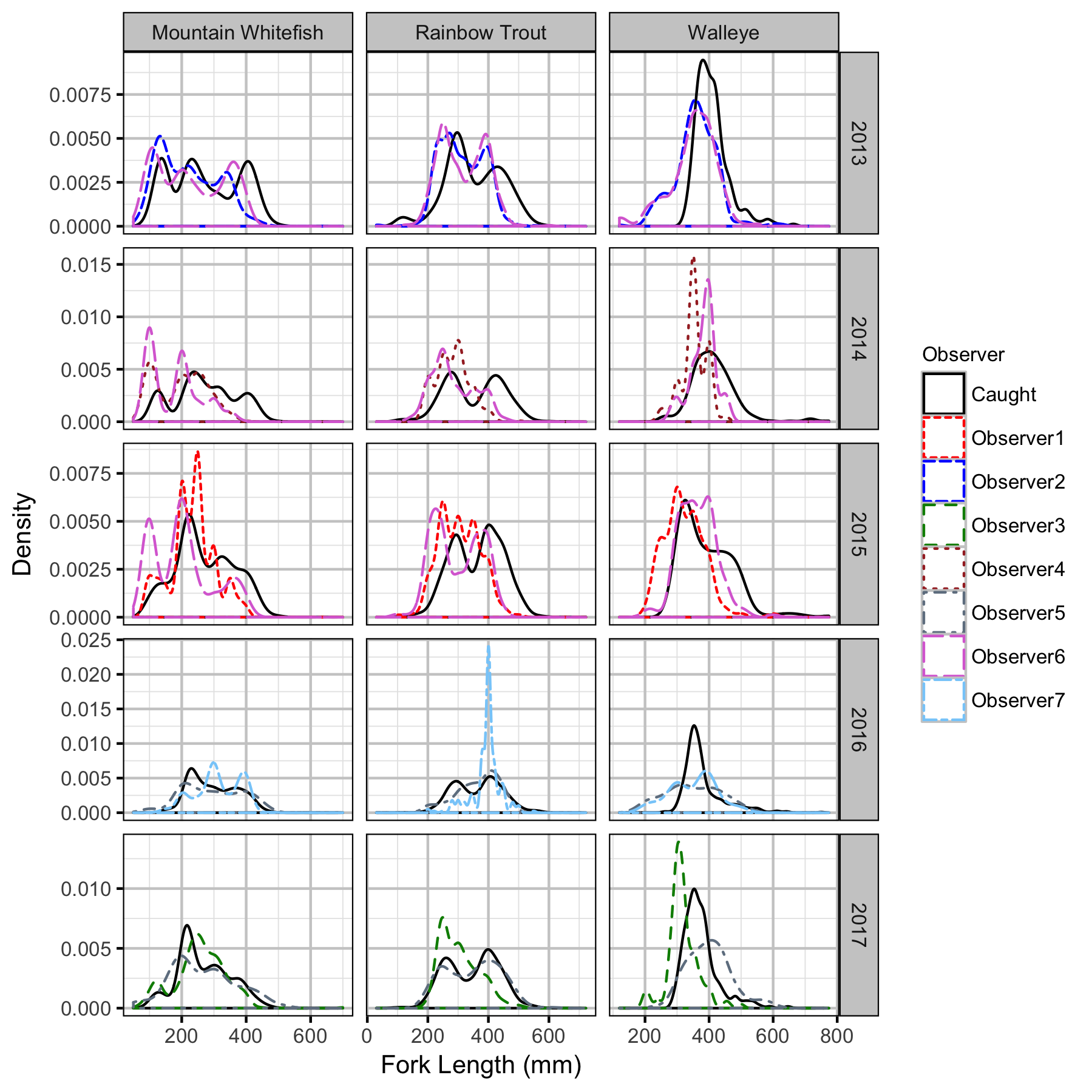Figure 23. Uncorrected length density plots by species, year and observer.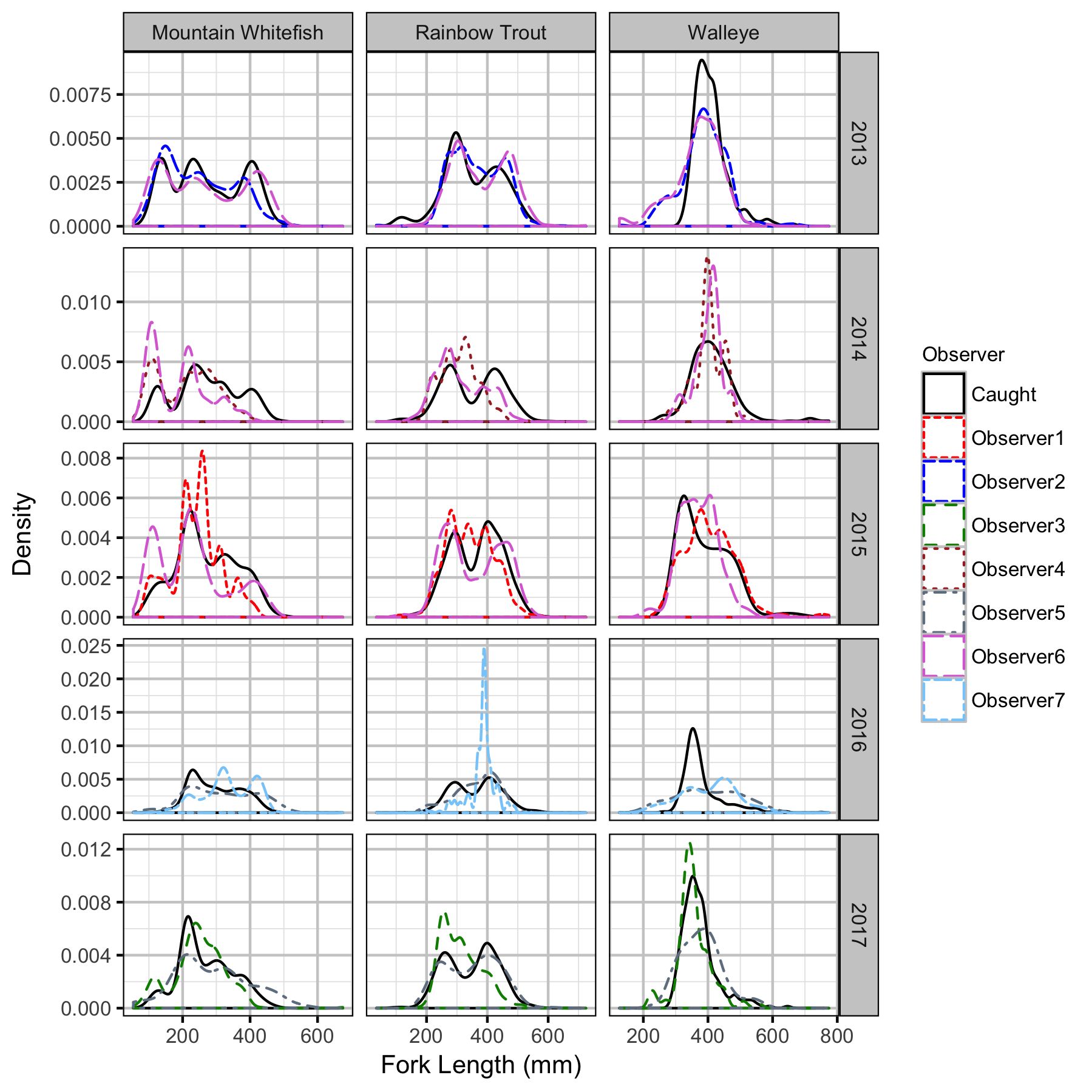Figure 24. Corrected length density plots by species, year and observer.

### Capture Efficiency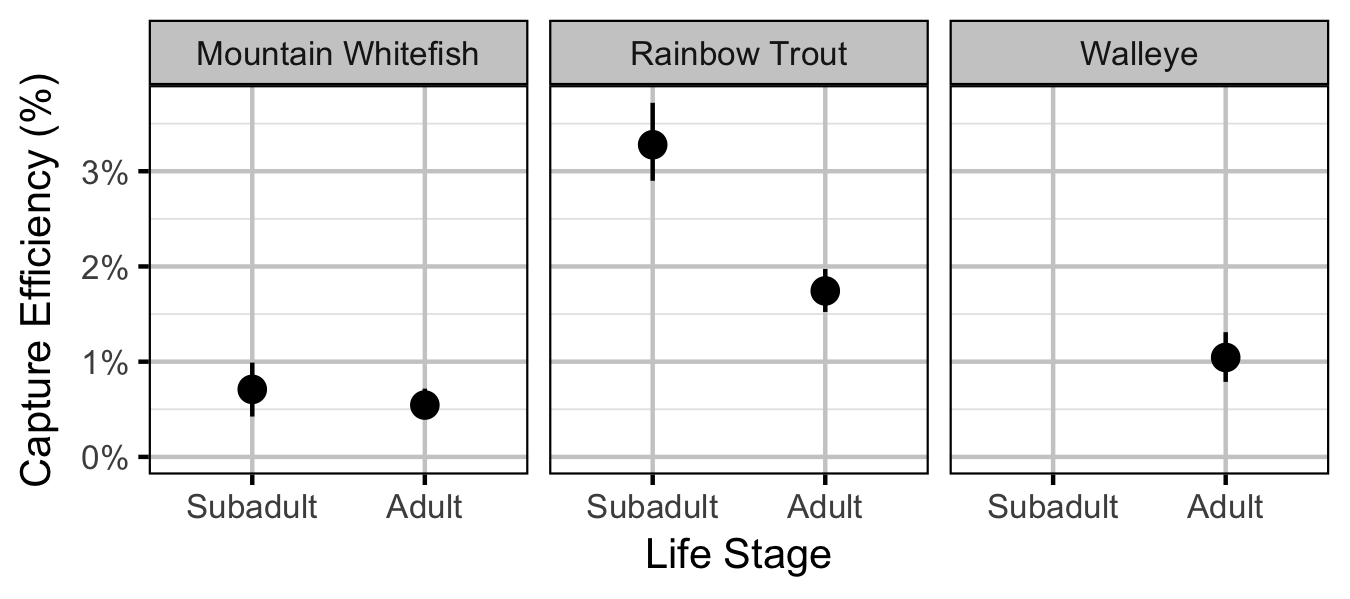Figure 25. Predicted capture efficiency by species and life stage (with 95% CRIs).

### Abundance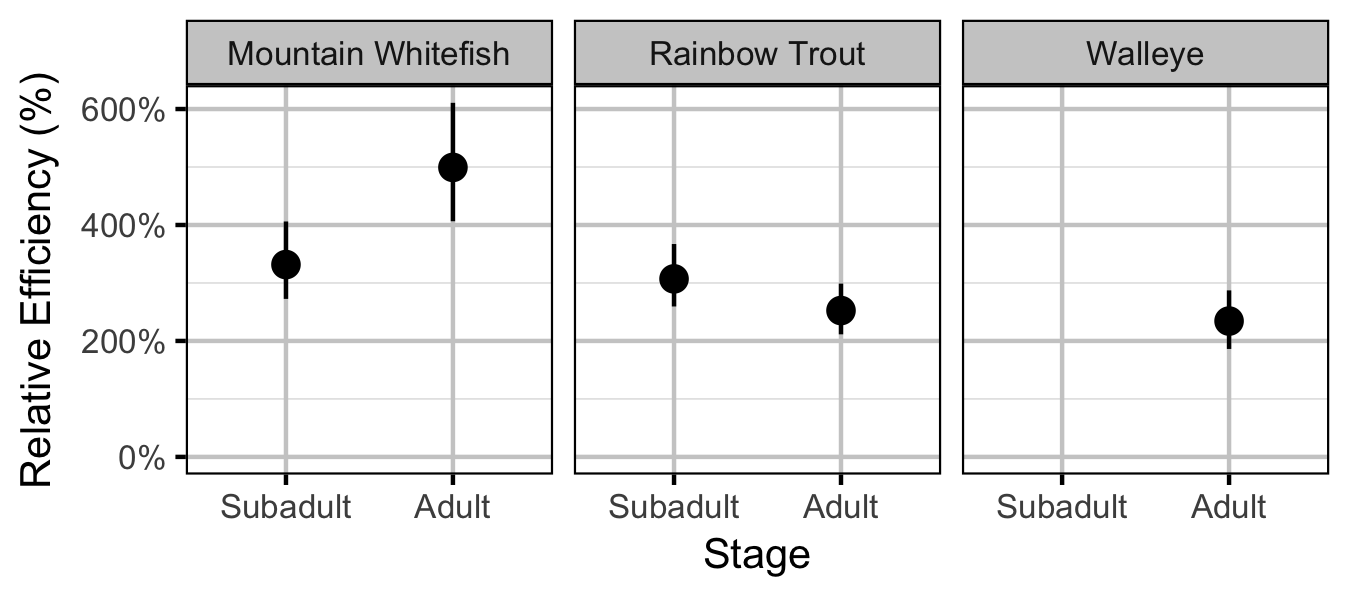Figure 31. Effect of counting (versus capture) on encounter efficiency by species and stage (with 95% CIs).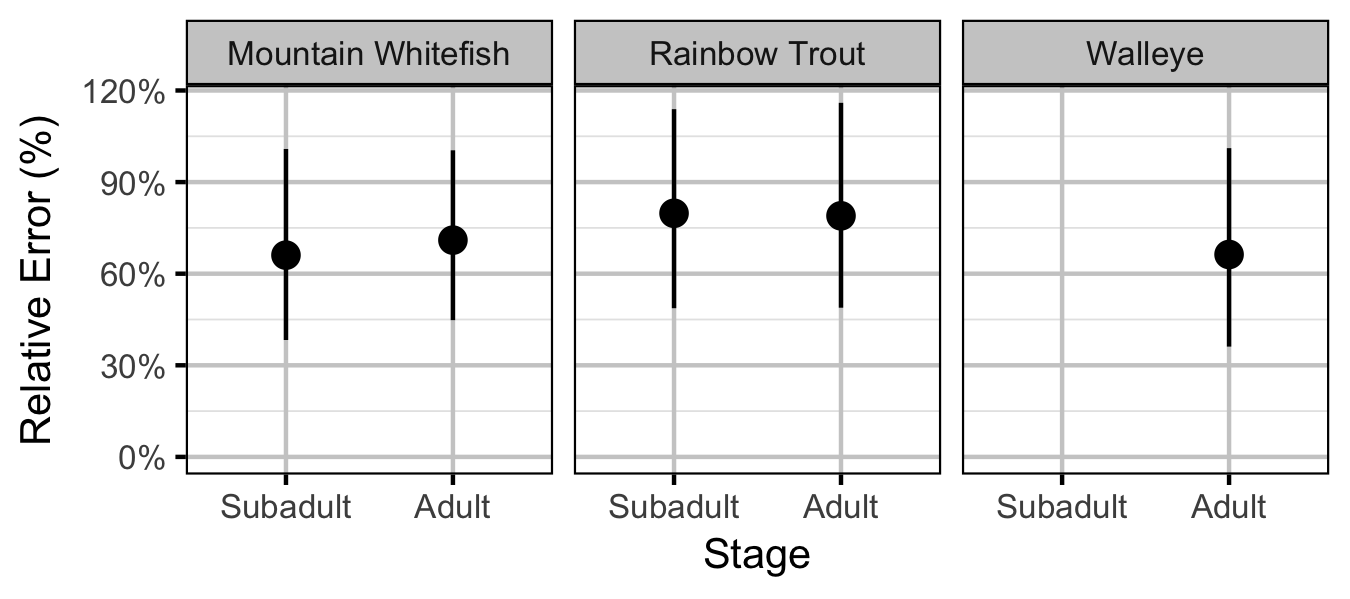Figure 32. Effect of counting (versus capture) on overdispersion by species and stage (with 95% CIs).

### Stock-Recruitment

#### Mountain Whitefish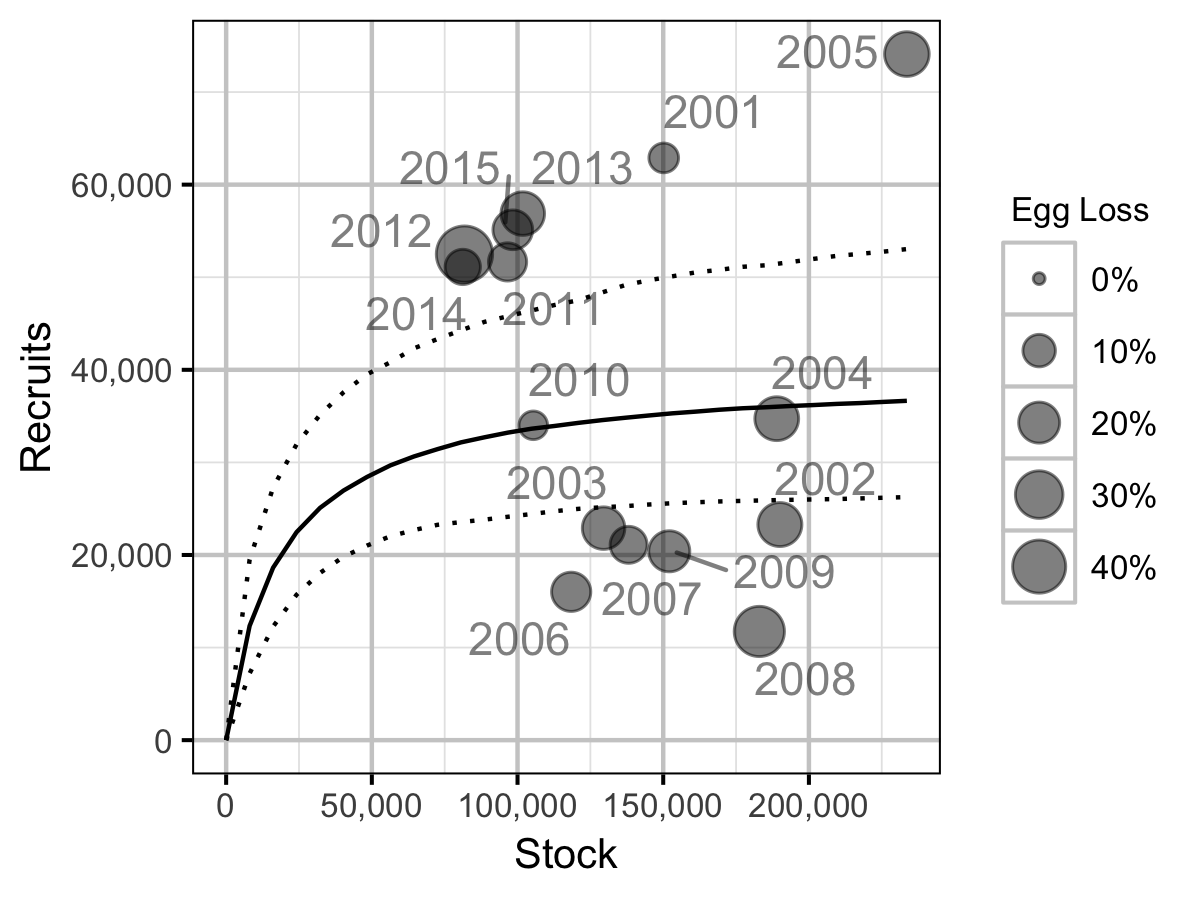Figure 43. Predicted stock-recruitment relationship from Age-2+ spawners to subsequent Age-1 recruits by spawn year (with 95% CRIs).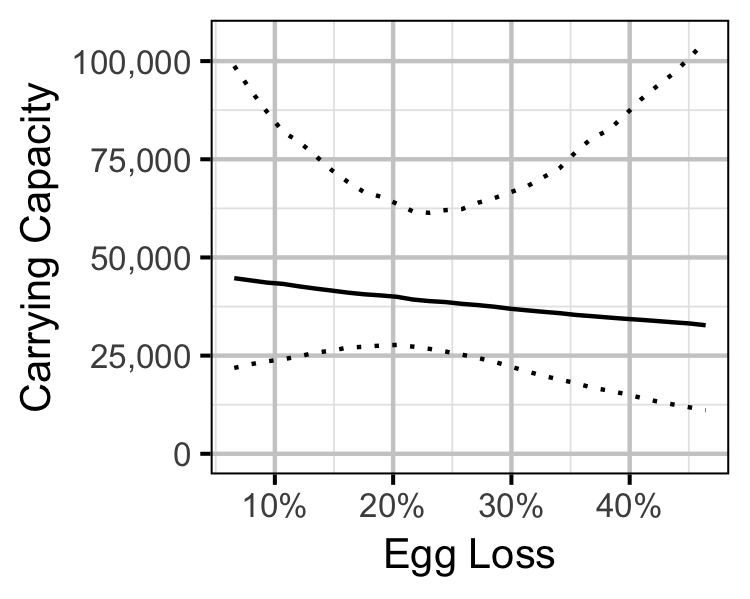Figure 44. Predicted Age-1 recruits carrying capacity by percentage egg loss (with 95% CRIs).

#### Rainbow Trout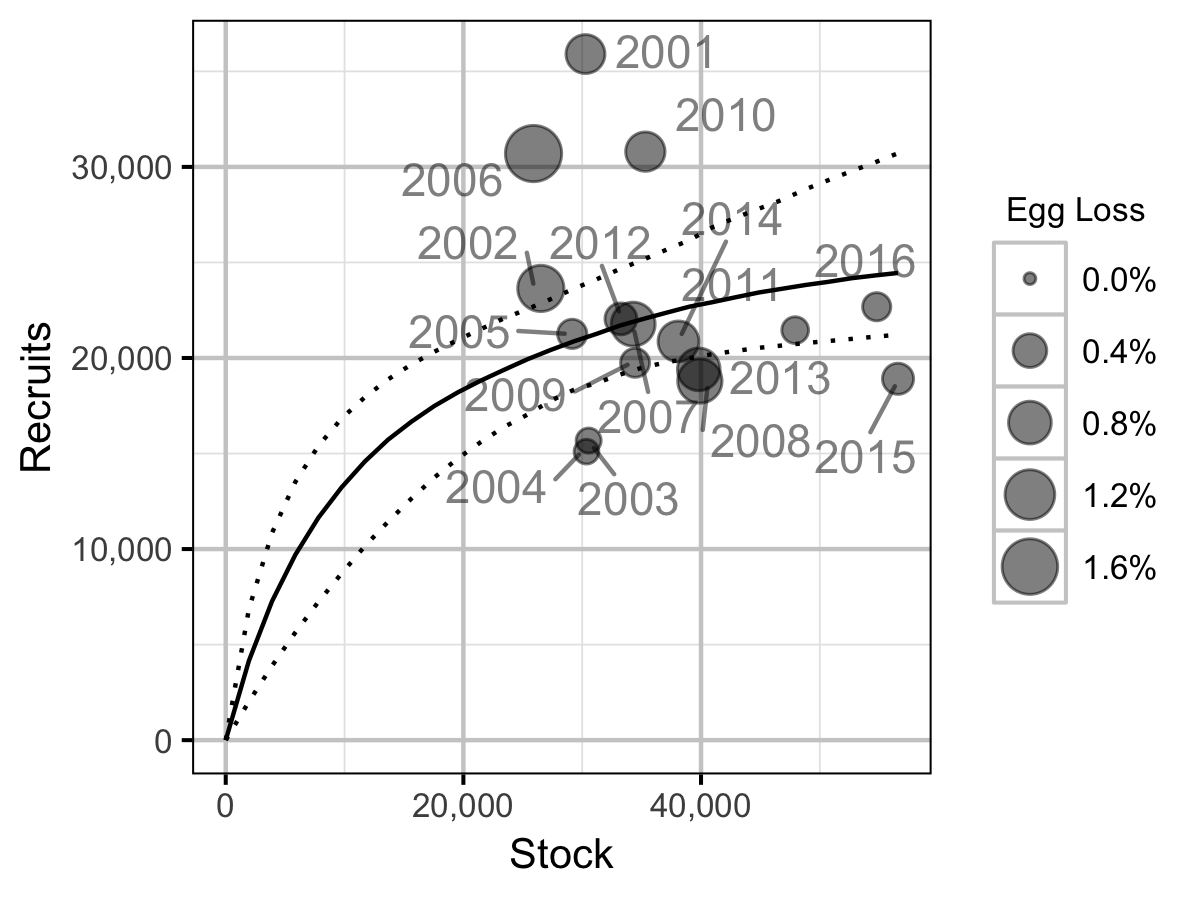Figure 45. Predicted stock-recruitment relationship from Age-2+ spawners to subsequent Age-1 recruits by spawn year (with 95% CRIs).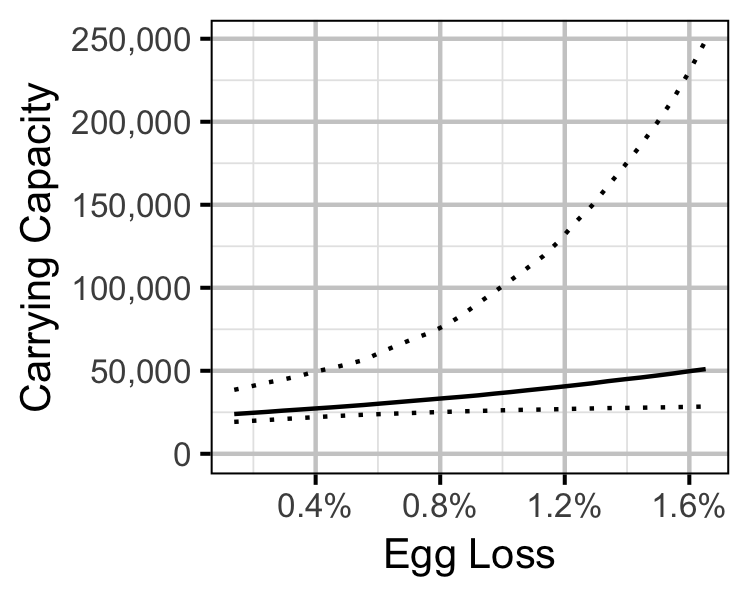Figure 46. Predicted Age-1 recruits carrying capacity by percentage egg loss (with 95% CRIs).

### Scale Age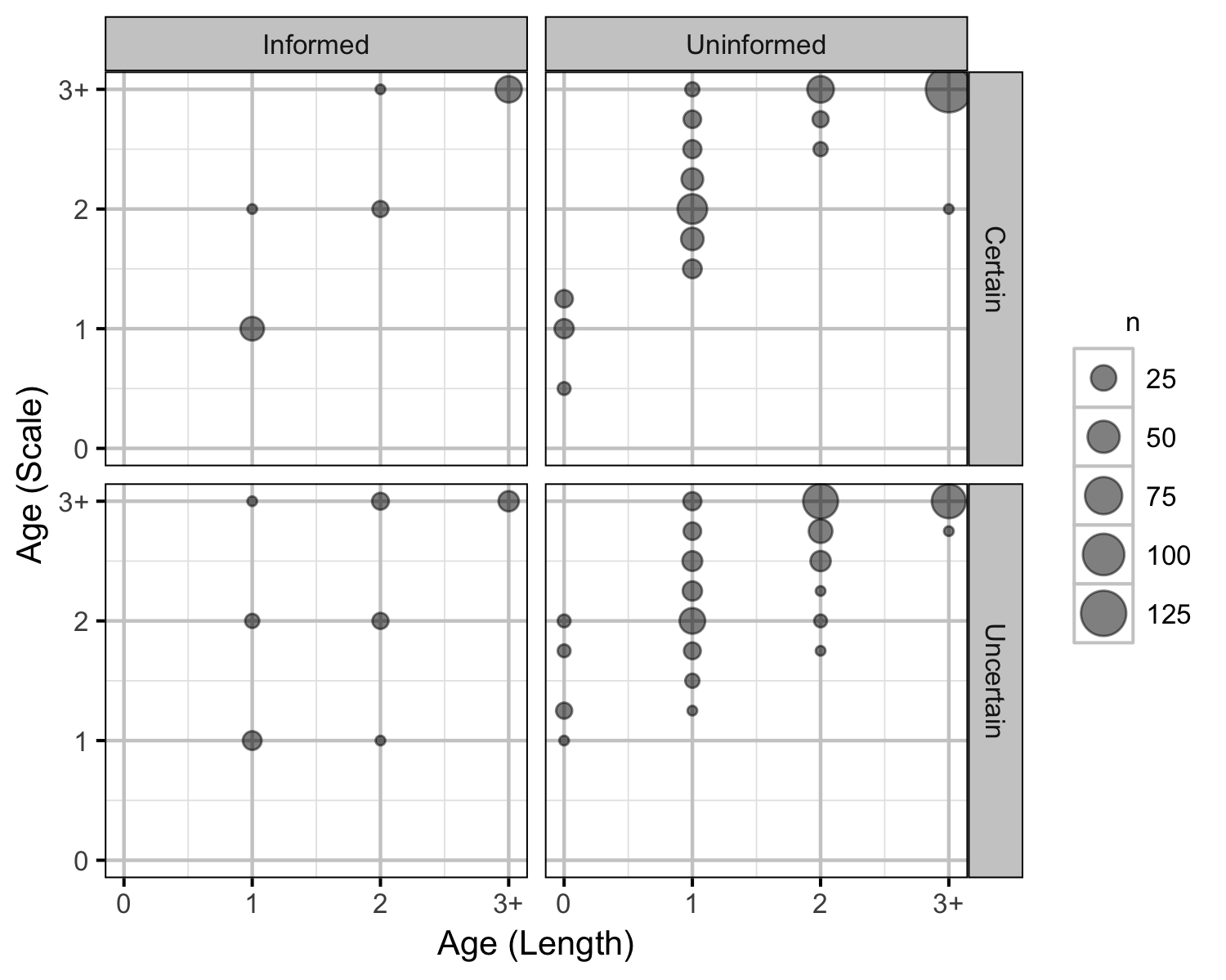Figure 47. Scaled based ages by length based ages by agers.